# AP Physics 2 : Other Fluid Dynamics

## Example Questions

### Example Question #1 : Other Fluid Dynamics

Viscosity is defined loosely as internal friction in a fluid. Which of the following fluids would you expect to have the highest viscosity?

Water

Molasses

Gasoline

Air

Molasses

Explanation:

The correct answer is molasses. Since this liquid is notably thicker and more difficult to move, it is intuitively sensed that is has the highest viscosity of this group of fluids.

### Example Question #51 : Fluid Dynamics

An object with mass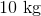and volume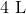and reference area of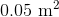is sinking in water with a constant velocity of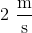. What is the drag coefficient of the object?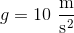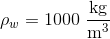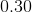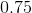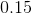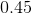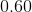Explanation:

We will start with Newton's second law: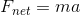Since the block is sinking at a constant velocity, we know that the net force is 0: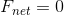There are three forces acting on the ball: gravitational, buoyant, and drag. If we designate any downward force as positive, we get: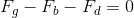(1)

Where: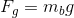(2)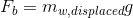Where: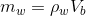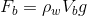(3)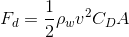(4)

Plugging expressions 2, 3, and 4 into 1, we get: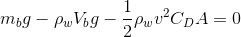Now we need to rearranging for the drag coefficient: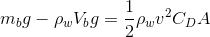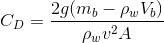Plugging in our values, we get: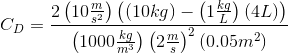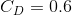### Example Question #52 : Fluid Dynamics

A spherical ball of mass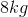and radius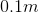is sinking in water. What is the terminal velocity of the ball?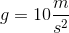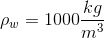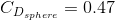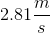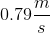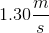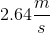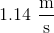Explanation:

We will start with Newton's second law for this problem:When the ball reaches terminal velocity, we know that the net force on the ball is equal to 0:There are three forces acting on the ball: gravitational, buoyant, and drag. If we designate any downward force as positive, we get:(1)

Where:(2)Where: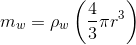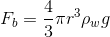(3)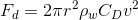(4)

Substituting expressions (2), (3), and (4) into (1), we get: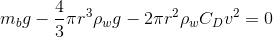Now we just need to rearranging for velocity.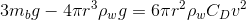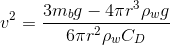We have all of these values, so time to plug and chug: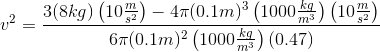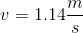### All AP Physics 2 Resources